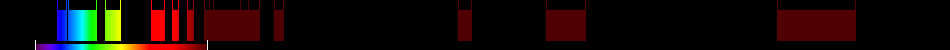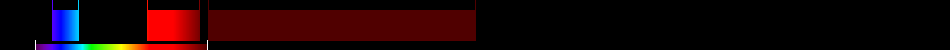# Information for Sensor and Index

## Formula

General Formula $\frac{{\mathrm{NIR}}-{\mathrm{RED}}-{y}\left({\mathrm{RED}}-{\mathrm{BLUE}}\right)}{{\mathrm{NIR}}+{\mathrm{RED}}-{y}\left({\mathrm{RED}}-{\mathrm{BLUE}}\right)}$ $\frac{{9}-{5}-{y}\left({5}-{1}\right)}{{9}+{5}-{y}\left({5}-{1}\right)}$ Automatic

## Sensor

Name Sentinel-2A 13 433-2280 10-60 98.6 ESA 2015-06-23 yes

## Index

Name Atmospherically Resistant Vegetation Index ARVI $\frac{{\mathrm{NIR}}-{\mathrm{RED}}-{y}\left({\mathrm{RED}}-{\mathrm{BLUE}}\right)}{{\mathrm{NIR}}+{\mathrm{RED}}-{y}\left({\mathrm{RED}}-{\mathrm{BLUE}}\right)}$ NIR = [781:1399] y = quotient derived from the components of atmospheric reflectance in the blue and red channel (see literature, e.g. Bannari 1992, for details) 420:480,640:760,781:1399 Original formula Minimizes the effects of atmospheric scattering in comparison to the NDVI

## References